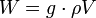# Buoyancy

Buoyancy is the upward force exerted on an object that is partially or completely immersed in a fluid. Since Archimedes was the first person to describe buoyancy, the fundamental description of buoyancy is called Archimedes' principle.

The force is a direct consequence of gravity and can be easily demonstrated. If we consider the forces on an arbitrary portion of still fluid in a gravity field, we can say that it is dragged downward by its weight W:$W = g \cdot \rho V$

where g is the gravitational acceleration, ρ is the fluid density and V is the volume of fluid.

For the fluid to remain still, the sum of all exerting forces must equal zero:$\sum F_i=0$

To achieve this condition, an upward force of the same intensity as the weigth must be exerted. This force is actually the sum of all pressure forces on the surface of the fluid portion.

If we substitute the above portion of fluid with a different body, the above relation still applies. Thus, if the density of the body is less than the fluid's one, the body will float to a position in which the weight of the displaced water equals its own weight.

If the density of the body exceeds the fluid's one, the body will move downward and ultimately sink.

In the theoretical case of equal densities, the body will stay still in its initial position, not moving upward or downward.

## Nautical applications

As the force on a floating body equals the weight of the displaced fluid, the weight of ships is usually referred to as displacement, measured in long tons (2240 pounds/1017 kilograms]].

A cube of steel, weighing one long ton, has no buoyancy and will sink. If that same ton of steel is shaped to enclose a volume of air whose buoyancy is greater than one long ton, the vessel will float.Some content on this page may previously have appeared on Citizendium.